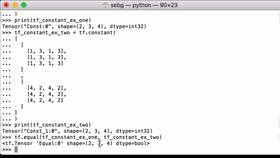This Lesson is for subscribersUnlock this lesson NOW!
• Get expert advice on how to TensorFlow Equal: Compare Two Tensors Element Wise
• Learn Deep Learning Technology Like Your Career Depends On It!

TensorFlow Equal: Compare Two Tensors Element Wise

TensorFlow Equal - Compare two tensors element wise for equality

Type: PRO     Duration: 5:08   Technologies: TensorFlow, Python

Page Sections: Video  |  Code  |  Transcript

< > Code:

You must be a Member to view code

Access all courses and lessons, gain confidence and expertise, and learn how things work and how to use them.

Transcript:

This Transcript is for subscribersUnlock this lesson's Transcript NOW!
• Get expert advice on how to TensorFlow Equal: Compare Two Tensors Element Wise